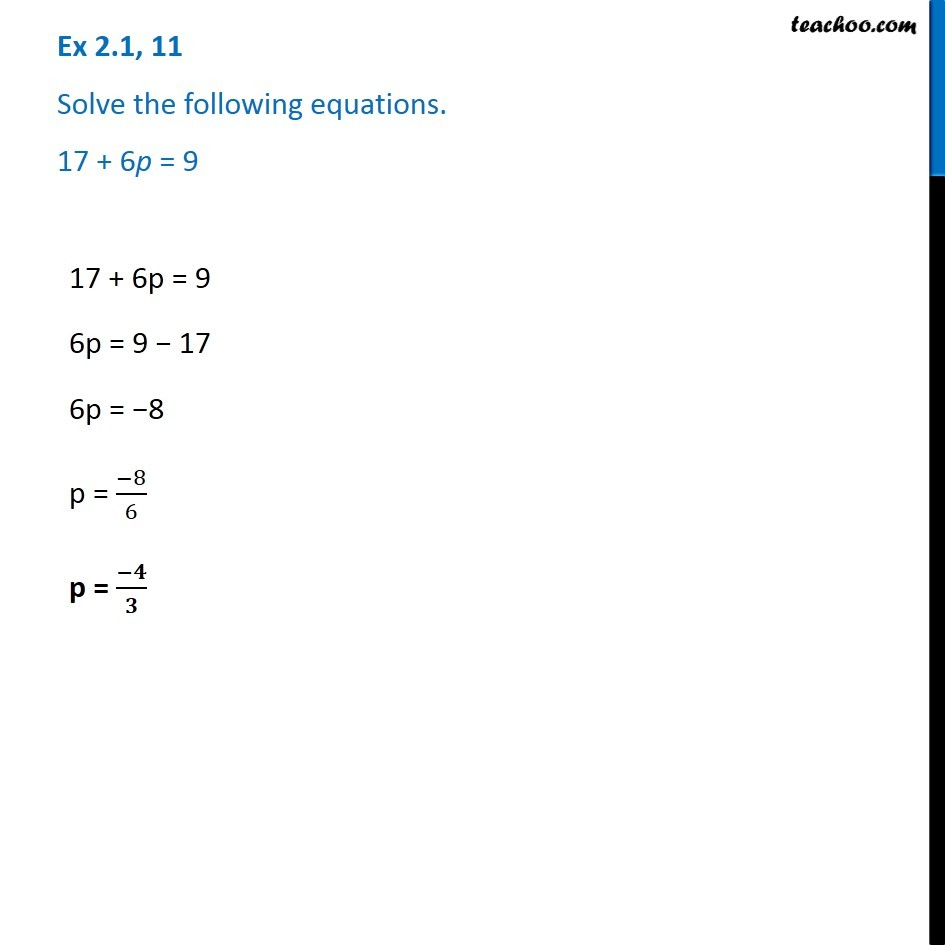Ex 2.1

Chapter 2 Class 8 Linear Equations in One Variable
Serial order wiseGet live Maths 1-on-1 Classs - Class 6 to 12

### Transcript

Ex 2.1, 11 - Chapter 2 Class 8 - Linear Equations in one variable - NCERT Book Solve the following equations. 17 + 6p = 9 17 + 6p = 9 6p = 9 − 17 6p = −8 p = (−8)/6 p = -4/3